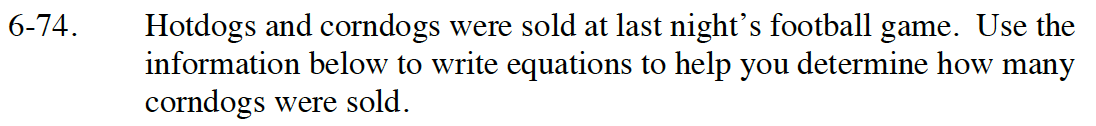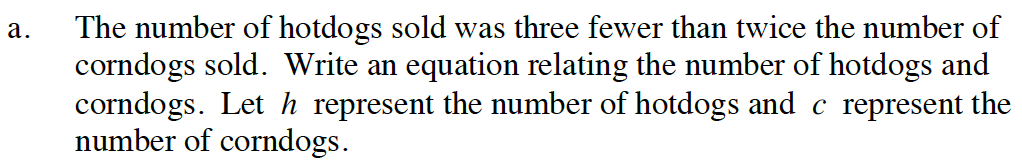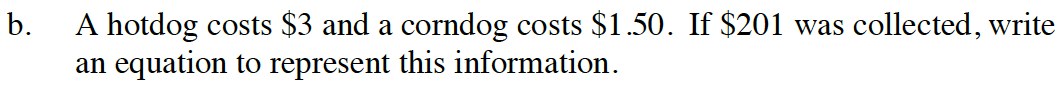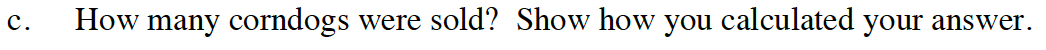Home > GB8I > Chapter cc46 > Lesson cc46.2.3 > Problem6-74

6-74.
1. Hotdogs and corndogs were sold at last night’s football game. Use the information below to write equations to help you determine how many corndogs were sold. Homework Help ✎

1. The number of hotdogs sold was three fewer than twice the number of corndogs sold. Write an equation relating the number of hotdogs and corndogs. Let h represent the number of hotdogs and c represent the number of corndogs.

2. A hotdog costs $3 and a corndog costs$1.50. If \$201 was collected, write an equation to represent this information.

3. How many corndogs were sold? Show how you calculated your answer.h = 2c − 3Multiply the number of hotdogs by 3 and the number of corndogs by 1.5.
Add the products and set them equal to 201.Substitute 2c − 3 from the equation in part (a) into the equation in part (b).

Solve for c.